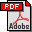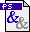Simple parallel algorithms for the replacement edge problem and related problems on minimum spanning trees Authors: Jyrki Katajainen and Jesper Larsson Träff Published in: Proceedings of the 18th Australasian Computer Science Conference, Australian Computer Science Communications 17,1, (1995), 254-261 Full text:PDF (141 kB)PS (244 kB) Abstract: Let G be a connected, undirected, and weighted graph with n vertices and m edges. Further, let S be a spanning tree of G and T a minimum spanning tree of G. In this paper we present parallel algorithms for solving the following problems: (a)  Given G and T, for each edge e of T, determine a replacement edge f by which e should be replaced to create a new minimum spanning tree if e is removed from G. (b)  Given G and T, find a most vital edge of G with respect to T, that is, an edge whose removal has the largest impact on the weight of T. (c)  Given G and S, determine whether S is a minimum spanning tree. (d)  Given G and T, compute, for each edge e of G, by how much the weight of e can change without affecting the minimality of T. The algorithms run in O(logn) time and O(m) space with m processors. The problems (a), (b), and (d) are solved on a MINIMUM CRCW PRAM, whereas the problem (c) is solved on a CREW PRAM. The algorithms utilizes a simple technique for computing functions defined on paths in rooted trees that might be of independent interest. BibLATEX: ```@inproceedings{KT1995C, author = {Jyrki Katajainen and Jesper Larsson Tr\"{a}ff}, title = {Simple parallel algorithms for the replacement edge problem and related problems on minimum spanning trees}, booktitle = {Proceedings of the 18th Australasian Computer Science Conference}, series = {Australian Computer Science Communications}, volume = {17}, number = {1}, year = {1995}, pages = {254--261}, } ```